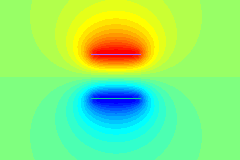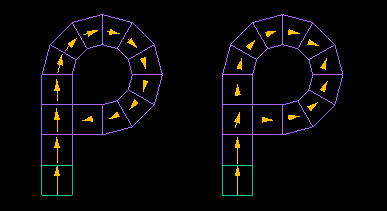The "FEM" is a widely used method for electromagnetic field analysis.
The FEM is based on differential equations and solves simultaneous equations assuming that the interaction of bodies is transmitted from one neighbor to another, including in space.
Various products are available from many vendors, and this analysis method is used universally.
However, the "FEM" is not suitable for the following problems, since the space must be Mesh Division with all the bodies in the region to be analyzed.
Open domain problems (setting boundary conditions) such as those involving infinite space.
Problems such as multiple bodies moving in space (twisted spatial mesh)

On the other hand, the "Magnetic Moment Method," "Surface Charge Method," and "Boundary Element Method" are examples of fast methods that do not require a spatial mesh.
These are analytical methods based on integral equations and are a type of " Integral Equation Method".

The Magnetic Moment Method and Surface Charge Method are physics-based methods that use physical quantities such as magnetic moment and surface charge as variables to calculate the direct interaction of distant bodies and solve them using simultaneous equations.Calculation of the magnetic field produced by a magnet using the "Magnetic Moment Method". (calculation time less than 1 second)Calculation of electrode potential using the "surface charge method". (calculation time less than 1 second)

Although these methods are much faster than the FEM, each has significant drawbacks that have made their practical application difficult.
For example, the " Boundary Element Method" cannot handle nonlinear problems such as magnetic saturation.
The "Magnetic Moment Method" and "Surface Charge Method" are not always applicable to magnetic and dielectric materials.

To solve this problem correctly, we gave independent variables to the polarized magnetic charges of all element surfaces in the "Magnetic Moment Method.
We then named them polarized magnetic charge elements.

We have also developed (eddy) current elements that can also calculate eddy and induced currents.

These polarized magnetic charge elements, (eddy) current elements, and surface charge elements are combined and named the " Integral Element Method.

The following are examples of problems that cannot be correctly calculated using the Magnetic Moment Method or the Surface Charge Method, but can be correctly calculated using the Integral Element Method.On the left is the "Magnetic Moment Method" and on the right is the "ELF's original Integral Element Method" (polarized magnetic charge elements).
The green element at the bottom is the magnet, and the other purple elements are the magnetic elements.

The magnetic flux sent from one magnet element at the bottom goes straight and draws a loop in the Magnetic Moment Method.
In ELF's method, the magnetic flux also branches to the right and solves the problem correctly.On the left is the " Surface Charge Method" and on the right is the " ELF's original integral element method" (polarized charge elements).

There are two ring-shaped dielectrics with a relative permittivity of 20, and red and green linear (flat) electrodes on the top and bottom.
The electrodes are given positive and negative potentials to apply electric fields to the dielectrics.

In the " Surface Charge Method," there are no polarization vectors inside the elements, so the electric flux does not pass through the interior of the dielectric, and are immediately emitted into space.
ELF's method solves the problem correctly.

In summary, the "Integral Element Method" has the following features
・Faster than the "FEM" method
・Easy to handle because spatial mesh and boundary conditions are not required
・Spatial mesh is not distorted by the motion of multiple bodies
・Highly accurate solutions for spatial electric/magnetic fields can also be obtained using analytical formulas
・Easy mixing of different scale parts
・Easy analysis including magnets
・This method can also be used for problems that cannot be solved correctly by the Magnetic Moment Method or Surface Charge Method
・Charged-particle trajectory calculations do not require a spatial mesh, and electric and magnetic fields are calculated directly from the source, so they are highly accurate

a:5054 t:2 y:0